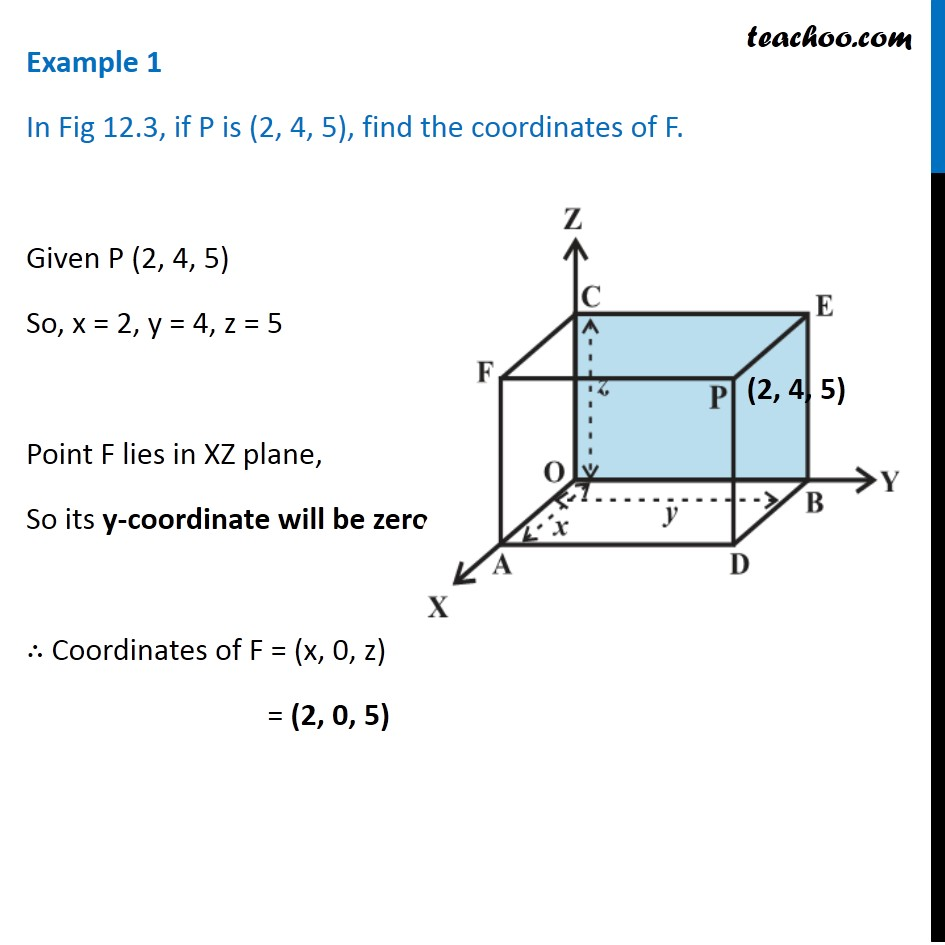Examples

Chapter 11 Class 11 - Intro to Three Dimensional Geometry
Serial order wiseLearn in your speed, with individual attention - Teachoo Maths 1-on-1 Class

### Transcript

Example 1 In Fig 12.3, if P is (2, 4, 5), find the coordinates of F. Given P (2, 4, 5) So, x = 2, y = 4, z = 5 Point F lies in XZ plane, So its y-coordinate will be zero ∴ Coordinates of F = (x, 0, z) = (2, 0, 5)